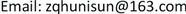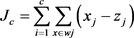﻿ 基于因子分析和聚类的客户细分研究 Customers Segmentation Based on Factor Analysis and Cluster

E-Commerce Letters
Vol. 08  No. 02 ( 2019 ), Article ID: 30099 , 10 pages
10.12677/ECL.2018.82007

Customers Segmentation Based on Factor Analysis and Cluster

Qihui Zhang

College of Economics and Management, Shandong Jiaotong University, Jinan ShandongReceived: Apr. 16th, 2019; accepted: Apr. 30th, 2019; published: May 7th, 2019ABSTRACT

In order to individual customer relationship maintenance, this paper selects auction customers as the research object, using the method of factor analysis to summarize the eleven evaluation index as five evaluation indexes that are more easy to explain and comprehensive evaluation of customer and clustering are based on factor analysis. Aiming at the blindness set the number of clusters, the quality clustering criterion function to measure the number of clusters is put forward. Through calculation and analysis of the actual data, the experimental results show that the number of customer clusters is more ideal based on factor analysis and clustering criterion function. The clustering results has better explain ability and scientific customer segmentation ability on customer bidding behavior.

Keywords:Factor Analysis, Cluster Analysis, Customer SegmentationCopyright © 2019 by author(s) and Hans Publishers Inc.

This work is licensed under the Creative Commons Attribution International License (CC BY).

http://creativecommons.org/licenses/by/4.0/1. 引言

2. 客户评价指标

3. 因子分析评价法

$\begin{array}{l}{x}_{1}={a}_{11}{F}_{1}+{a}_{12}{F}_{2}+{a}_{13}{F}_{3}+\cdots +{a}_{1m}{F}_{m}+{\epsilon }_{1}\\ {x}_{2}={a}_{21}{F}_{1}+{a}_{22}{F}_{2}+{a}_{23}{F}_{3}+\cdots +{a}_{2m}{F}_{m}+{\epsilon }_{2}\\ {x}_{3}={a}_{31}{F}_{1}+{a}_{32}{F}_{2}+{a}_{33}{F}_{3}+\cdots +{a}_{3m}{F}_{m}+{\epsilon }_{3}\\ ⋮\\ {x}_{P}={a}_{P1}{F}_{1}+{a}_{P2}{F}_{2}+{a}_{P3}{F}_{3}+\cdots +{a}_{Pm}{F}_{m}+{\epsilon }_{P}\end{array}$

1) 确认待分析的数据变量是否适合因子分析；

2) 进行初步因子分析，选取因子变量；

3) 因子旋转和对因子变量进行解释；

4) 计算因子得分和综合得分进行进一步分析。

4. 在线竞拍客户评价

4.1. 原始数据的标准化

4.2. KMO和Bartlett检验

4.3. 提取公共因子变量

4.4. 公共因子变量的命名及解释

4.5. 计算因子得分

${F}_{ij}={w}_{j1}{x}_{i1}+{w}_{j2}{x}_{i2}+{w}_{j3}{x}_{i3}+\cdots +{w}_{jp}{x}_{ip}\left(j=1,2,3,\cdots ,m\right)$

Fij为第i行样本在第j个因子上的得分，wjp为第j个因子在第p个变量处的因子得分系数，xip为第i行样本的第p个变量标准化后的数据，因此根据标准化后的指标数据(表5)和成份得分系数矩阵(表6)就可以计算出各样本数据在各因子上的得分(表7)。Table 7. Customer factor score and comprehensive score table

4.6. 计算综合得分

$Z{F}_{1}=\left(\begin{array}{l}0.35787*0.2008\text{}+0.18592*\left(-0.5221\right)+0.12282*\\ \left(-0.0625\right)+0.09253*-0.7702+0.09152*\left(-0.3327\right)\end{array}\right)/0.85066=-0.1582$

5. 客户聚类实证

5.1. 相识性度量方法-距离

$d\left({x}_{i},{x}_{j}\right)=\sqrt{{\left({x}_{i1}-{x}_{j1}\right)}^{2}+\cdots +{\left({x}_{ip}-{x}_{jp}\right)}^{2}}$

5.2. 聚类准则函数

${Z}_{j}=\frac{1}{n}\underset{j=0}{\overset{n}{\sum }}{x}_{j}$

${J}_{c}=\underset{i=1}{\overset{c}{\sum }}\underset{x\in wj}{\sum }\left({x}_{j}-{z}_{j}\right)$

5.3. C-均值聚类算法

C-均值聚类算法是目前应用最广泛最成熟且快速简单的聚类分析方法，C-均值聚类算法的步骤为：

(1) 按最大距离原则选取C个初始聚类中心；

(2) 按最小距离原则将待分类对象逐个分到离它最近的C个类的某一类，并计算误差平方和Jc

(3) 重新计算分配后的各类心和误差平方和Jc

5.4. 客户聚类实证Table 8. Sum of squares of errors for different classificationsTable 9. Clustering results when the number of classifications is 4

5.5. 客户聚类解释

I类：低信誉客户。这类客户的拒拍率、流拍率和退拍率都较高。拒拍率、流拍率较高的客户多是自己有商品在拍卖，通过托拍来提高自己拍品的拍卖价格，由于无人追拍而使自己得拍，得拍后只能以流拍或拒拍的方式来结束拍卖，影响了正常的竞拍秩序。退拍率较高的客户对拍品品相要求严格，这类客户得拍后，可提醒卖家在发货前就拍品品相与买家多沟通。低信誉竞拍客户无论注册时间长短，活跃程度如何，由于真实成交少，对贡献利润低，对于低信誉客户如果得拍后拒绝交易可以扣除其一定的押金或进一步降低其信誉值或授权卖家将其拉入黑名单。

II类：一般价值客户。一些客户虽然注册网站时间较长，也有一些拍卖或零售成交记录，但现在很少登录网站或虽经常登录网站但较少参与竞拍或零售购买商品。还有一些客户注册时间不长，也不经常登录网站或虽经常登录网站但较少参与竞拍或零售购买商品。这些客户的特征是不管注册网站时间长短，登录频繁与否，现在较少参与竞拍或零售购买商品，这类客户可能受制于自身经济条件只关注喜欢的商品，对这类客户可以分析其以前的竞拍喜好，通过手机短信的方式欢迎用户常来网站看看和有针对性地推荐其可能喜欢的拍品。

III类：新价值客户。这类客户虽然注册时间不长，但经常登录网站参加竞拍或者以零售方式购买商品，累计拍卖成交或零售成交逐渐增大。这类客户是在线竞拍网站客户的新生力量，这类客户有的是真正喜欢收藏，有的则可能是一时兴趣，他们可能分化为稳定价值客户或一般价值客户。对这部分客户要实时跟踪，关注哪些客户可能转化为稳定价值客户，对其佣金可以根据其购买情况浮动管理，发展壮大这部分客户并使之转化为稳定价值客户是提升在线竞拍网站佣金收入的关键。

IV类：稳定价值客户。这类客户注册时间较长，经常登录网站参加竞拍或以零售方式购买商品，并且拒拍率、流拍率和退拍率较低，拍卖成交额或零售成交额都较大，这类客户是网站拍卖藏品的真正爱好者，有较强的购买欲望和购买能力，是在线竞拍网站佣金收入的主要来源，稳定这部分客户对维持在线竞拍网站的运营至关重要，可以降低其佣金比例或根据其成交额返回一定的佣金或赠送自制的有意义的纪念品。

6. 结语

Customers Segmentation Based on Factor Analysis and Cluster[J]. 电子商务评论, 2019, 08(02): 53-62. https://doi.org/10.12677/ECL.2018.82007

1. 1. 何媛. 基于客户关系管理中客户细分的研究与应用[J]. 现代营销, 2017(8): 53.

2. 2. 梁妮. 网络营销环境下的客户细分与营销策略[J]. 科技经济导刊, 2016(32): 247.

3. 3. 黄亚萍, 李垣江. 基于K-means算法的电子商务客户细分研究[J]. 电子设计工程, 2017, 25(2): 63-66.

4. 4. 马健悦. 我国城镇居民消费水平的聚类分析和因子分析[J]. 中国商论, 2018(2): 74-75.

5. 5. 麻雅静, 武刚. 基于因子分析与风险矩阵的IT外包风险评价[J]. 计算机工程, 2013, 39(9): 281-284.

6. 6. 卢辉. 数据挖掘与数据化运营实战思路、方法、技巧与应用[M]. 北京: 机械工业出版社, 2013: 130-131.

7. 7. 贺盛瑜, 马会杰, 滕喜华. 基于因子分析和聚类分析的我国电子商务发展水平研究[J]. 经济体制改革, 2017(2): 196-200.

8. 8. 曾小青, 徐秦. 基于消费数据挖掘的多指标客户细分新方法[J]. 计算机应用研究, 2013, 30(10): 44-47.

9. 9. 吴明礼, 黄亚非. 基于聚类的多指标客户细分方法[J]. 电脑知识与技术, 2018, 14(5): 18-21.